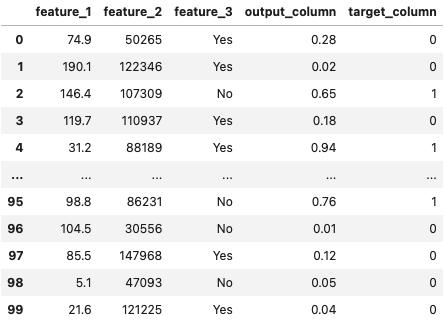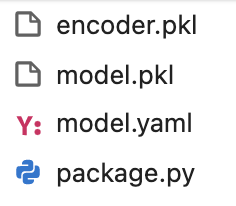To upload a model artifact to Fiddler, you need to package your model into a format that Fiddler understands.

A model package should contain:

# Model artifact

A model artifact is a serialized representation of your model as a Python object.

Artifacts can be stored in a variety of formats. Some include

• Pickle (`.pkl`)
• Protocol buffer (`.pb`)
• Hierarchical Data Format/HDF5 (`.h5`)

To start, place your model artifact in a new directory. This directory will be the model package you will upload to Fiddler.

# package.py script

Fiddler’s artifact upload process is framework-agnostic. Because of this, a wrapper script is needed to let Fiddler know how to interact with your particular model and framework.

The wrapper script should be named `package.py`, and it should be placed in the same directory as your model artifact. Below is an example of what `package.py` should look like.

``````from pathlib import Path
import pandas as pd

PACKAGE_PATH = Path(__file__).parent

class MyModel:

def __init__(self):
"""
Here we can load in the model and any other necessary
serialized objects from the PACKAGE_PATH.
"""

def predict(self, input_df):
"""
The predict() function should return a DataFrame of predictions
whose columns correspond to the outputs of your model.
"""

def get_model():
return MyModel()
``````

The only hard requirements for `package.py` are

• The script must be named `package.py`
• The script must implement a function called `get_model`, which returns a model object
• This model object must implement a function called `predict`, which takes in a pandas DataFrame of model inputs and returns a pandas DataFrame of model predictions

# model.yaml configuration file

You'll need to construct a YAML file with specifications for how your model operates. This can be easily obtained from fdl.ModelInfo() object.

### 📘Info

For information on constructing a fdl.ModelInfo() object, see Registering a Model.

Once you have your fdl.ModelInfo(), you can call its fdl.ModelInfo.to_dict() function to generate a dictionary that can be used for the YAML configuration file.

``````import yaml

with open('model.yaml', 'w') as yaml_file:
yaml.dump({'model': model_info.to_dict()}, yaml_file)
``````

Note that we are adding `model` key whose value is the dictionary produced by the `fdl.ModelInfo` object.

Once it’s been created, you can place it in the directory with your model artifact and `package.py` script.

# Preprocessing objects

The last component of your model package should be any serialized preprocessing objects that are used to transform the data before or after making predictions.

You can place these in the model package directory as well.

### 📘Info

For example, in the case that we have a categorical feature, we may need to encode it as one or more numeric columns before calling the model’s prediction function. In that case, we may have a serialized transform object called `encoder.pkl`. This should also be included in the model package directory.

# Example model package

Suppose we have the following dataset for which we've trained a model.We would like to write a `package.py` script for this model.

### 🚧Note

We will have to encode `feature_3` as numeric in order to run predictions on the data. For this, we have a serialized preprocessor (`encoder.pkl`) that we will load along with the model artifact.

Below is an example of how we might construct our `package.py` script.

``````%%writefile package.py

from pathlib import Path
import pandas as pd
import pickle

PACKAGE_PATH = Path(__file__).parent
MODEL_OUTPUTS = ['output_column']

class MyModel:

def __init__(self):

with open(PACKAGE_PATH / 'model.pkl', 'rb') as pkl_file:

# Load a categorical-to-numeric transformer
with open(PACKAGE_PATH / 'encoder.pkl', 'rb') as pkl_file:

def transform_input(self, input_df):

# Convert feature_3 from categorical to numeric
input_df['feature_3'] = self.encoder.transform(input_df[['feature_3']])

return input_df

def predict(self, input_df):

# Apply the transformations specified in transform_input
transformed_df = self.transform_input(input_df)

# Make predictions on the transformed data
predictions = self.model.predict(transformed_df)

# Store the predictions in a pandas DataFrame
prediction_df = pd.DataFrame(predictions, columns=MODEL_OUTPUTS)

return prediction_df

def get_model():
return MyModel()
``````

The final model package will look something like this:``````PROJECT_ID = 'example_project'